# Mathematical models of control systems

Evaluate the transfer function, G(s) = V0(s) / V(s) for the electric network shown in Figure (a).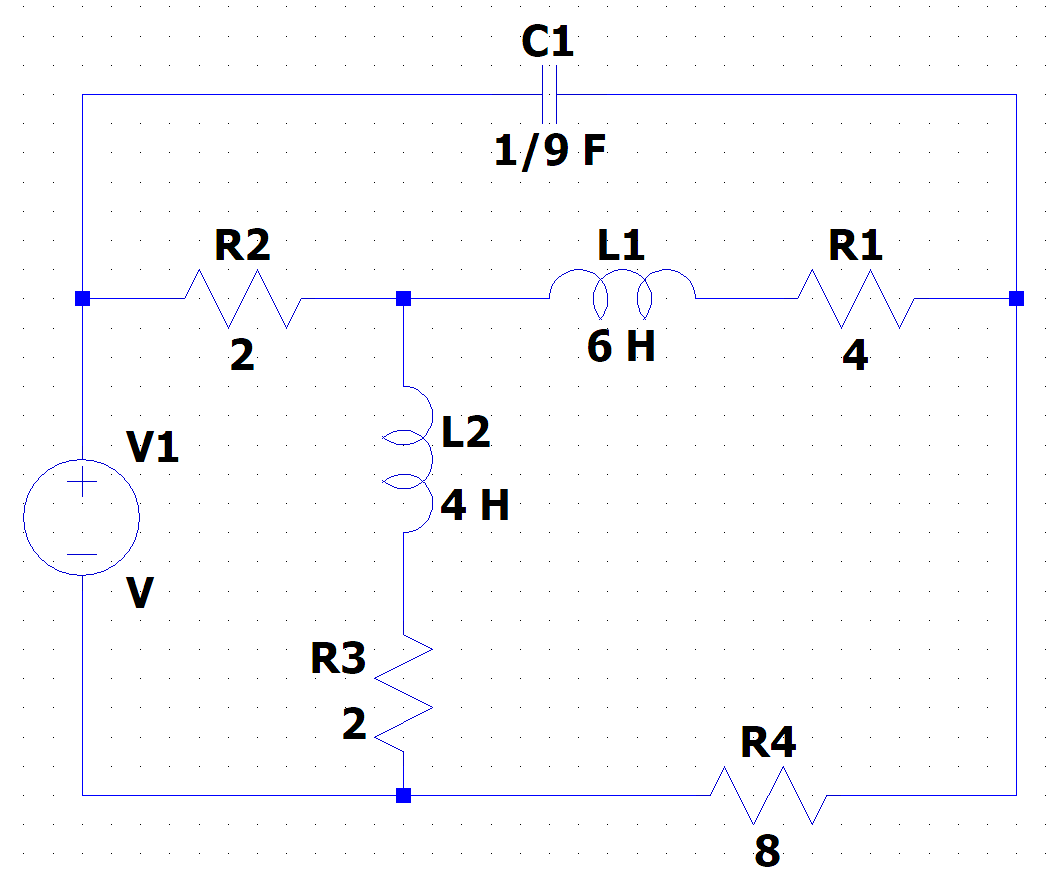We need at least 10 more requests to produce the answer.

0 / 10 have requested this problem solution

The more requests, the faster the answer.

All students who have requested the answer will be notified once they are available.

#### Earn Coin

Coins can be redeemed for fabulous gifts.

Similar Homework Help Questions
• ### ' 1. Review Question a) Name three applications for feedback control systems. b) Functionally, ho...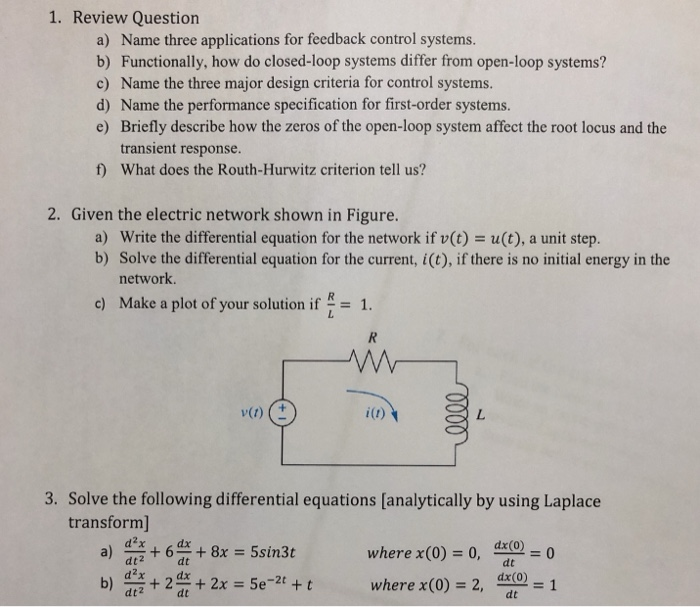' 1. Review Question a) Name three applications for feedback control systems. b) Functionally, how do closed-loop systems differ from open-loop systems? c) Name the three major design criteria for control systems. d) Name the performance specification for first-order systems. e) Briefly describe how the zeros of the open-loop system affect the root locus and the transient response. What does the Routh-Hurwitz criterion tell us? f) 2. Given the electric network shown in Figure. a) Write the differential equation for...

• ### Problem 4 17. Find the transfer function, G(s) = VL(s)/V(s), for each network shown in Figure...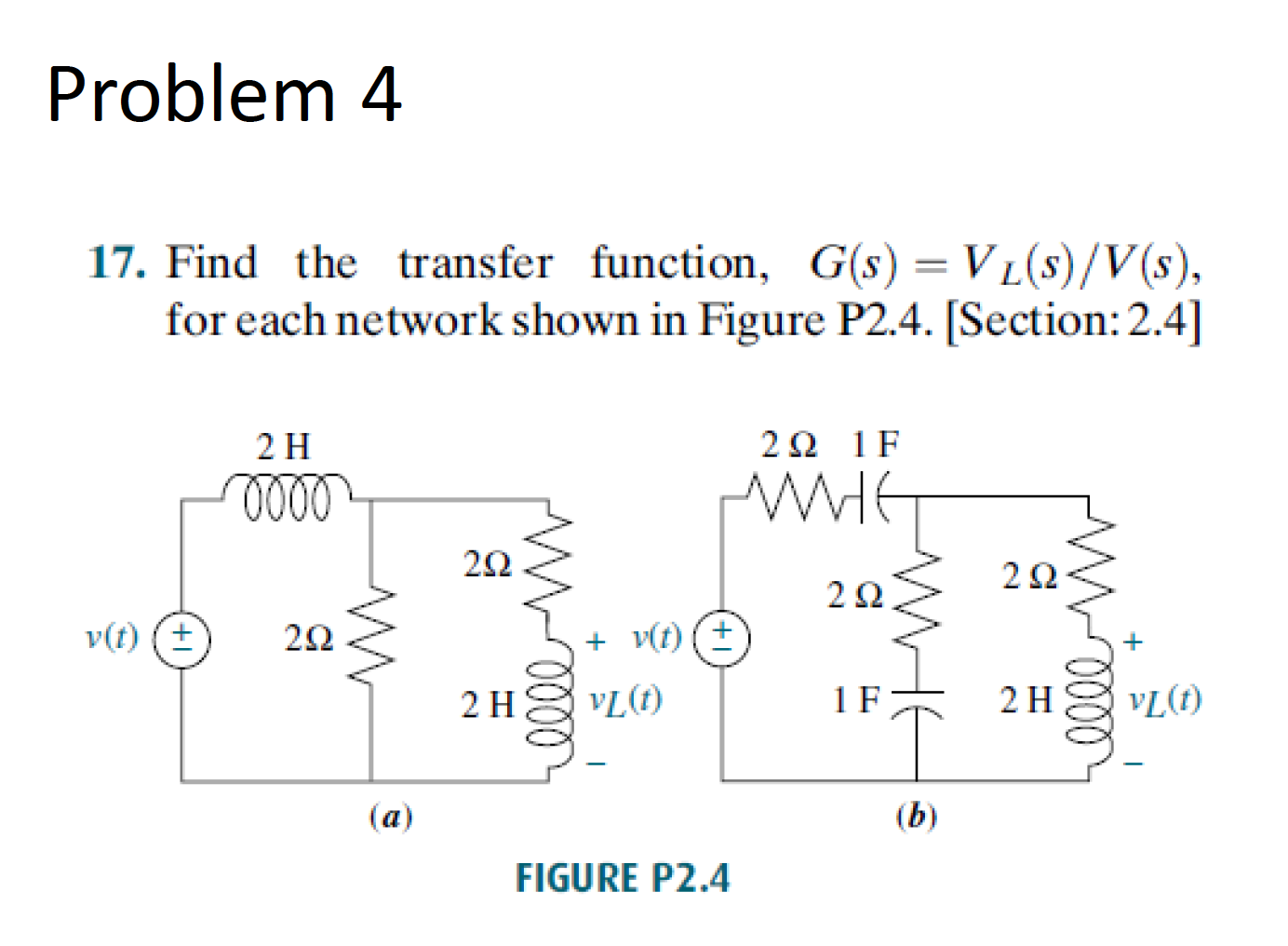Problem 4 17. Find the transfer function, G(s) = VL(s)/V(s), for each network shown in Figure P2.4. [Section: 2.4] 2 H 22 1F -1000 v(t) 203 v(t) (+) 2hvLT 21 vL (1) - (a) FIGURE P2.4

• ### Find the transfer function G(s)=Vo(s)/Vi(s) for the electrical network shown in the figure below. Express the...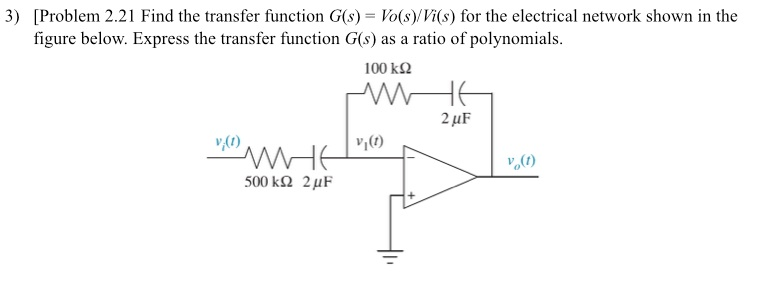Find the transfer function G(s)=Vo(s)/Vi(s) for the electrical network shown in the figure below. Express the transfer function G(s) as a ratio of polynomials.

• ### Find the transfer function G(s)=Vo(s)/Vi(s) for the electrical network shown in the figure below. Express the...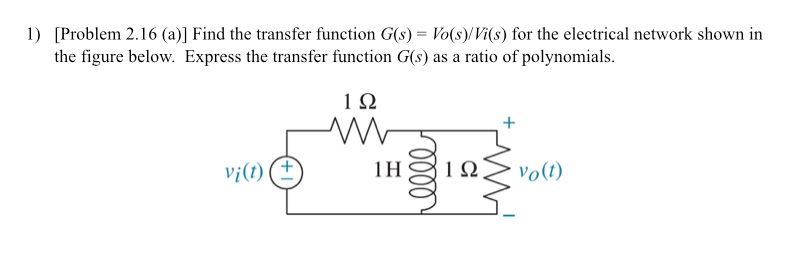Find the transfer function G(s)=Vo(s)/Vi(s) for the electrical network shown in the figure below. Express the transfer function G(s) as a ratio of polynomials.

• ### Answer all questions ELE380: Control Systems I Homework #1 Due: 10/3/2018 1. The circuit of a...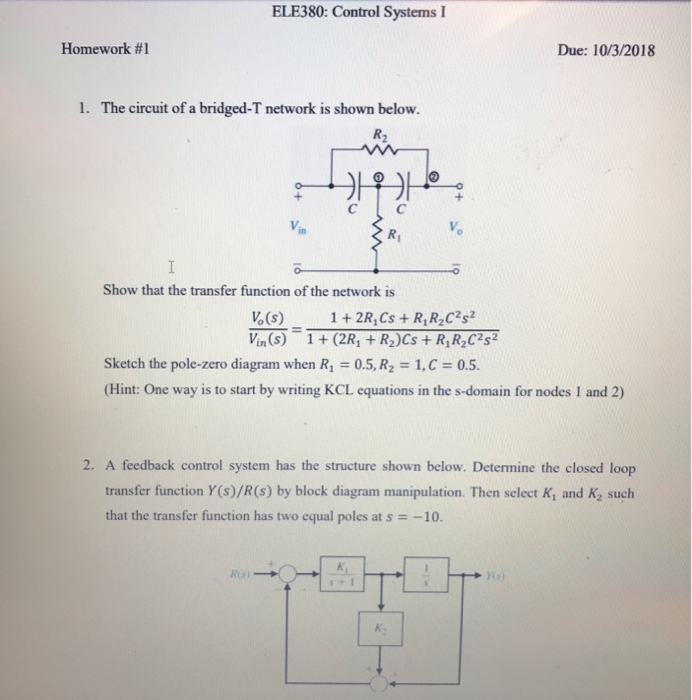Answer all questions ELE380: Control Systems I Homework #1 Due: 10/3/2018 1. The circuit of a bridged-T network is shown below. R2 CIC Show that the transfer function of the network is Vo (s)12R,Cs +R,R2C2s Vin(s) 1 (2R1 R2)Cs R,R2C2s2 Sketch the pole-zero diagram when R 0.5, R2 1,C 0.5 (Hint: One way is to start by writing KCL equations in the s-domain for nodes I and 2) 2. A feedback control system has the structure shown below. Determine the...

• ### Question 1 An electronic circuit is shown in Figure 1. In order to simulate the circuit,...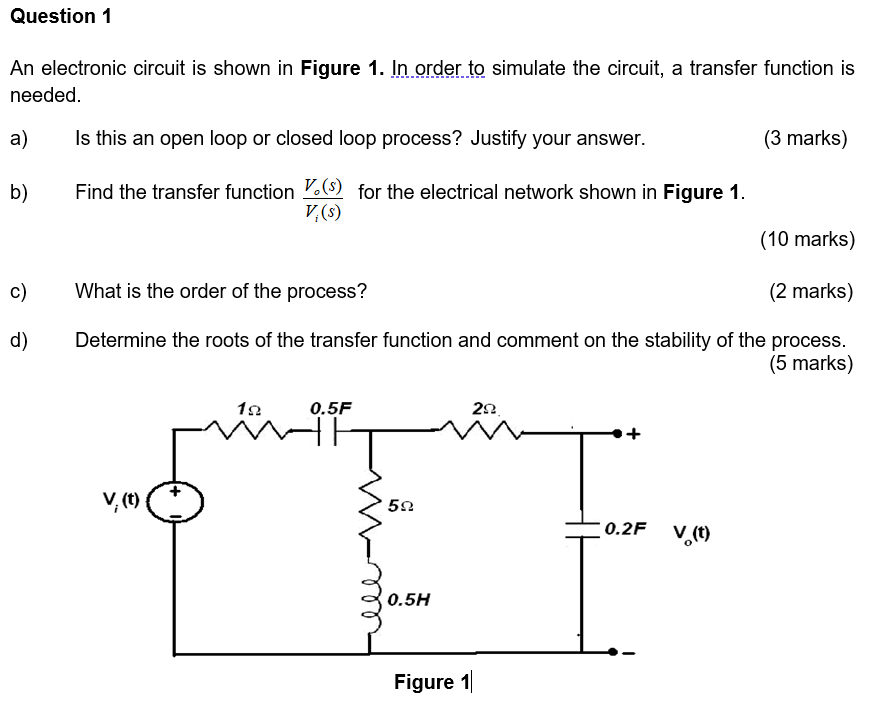Question 1 An electronic circuit is shown in Figure 1. In order to simulate the circuit, a transfer function is needed. a) Is this an open loop or closed loop process? Justify your answer. (3 marks) b) Find the transfer function V.(s) for the electrical network shown in Figure 1. V:(s) (10 marks) c) What is the order of the process? (2 marks) d) Determine the roots of the transfer function and comment on the stability of the process. (5...

• ### modern Control system (C6, CO4, PO2, PO3 and PO11 (a) A compensator is an additional component or circuit that i...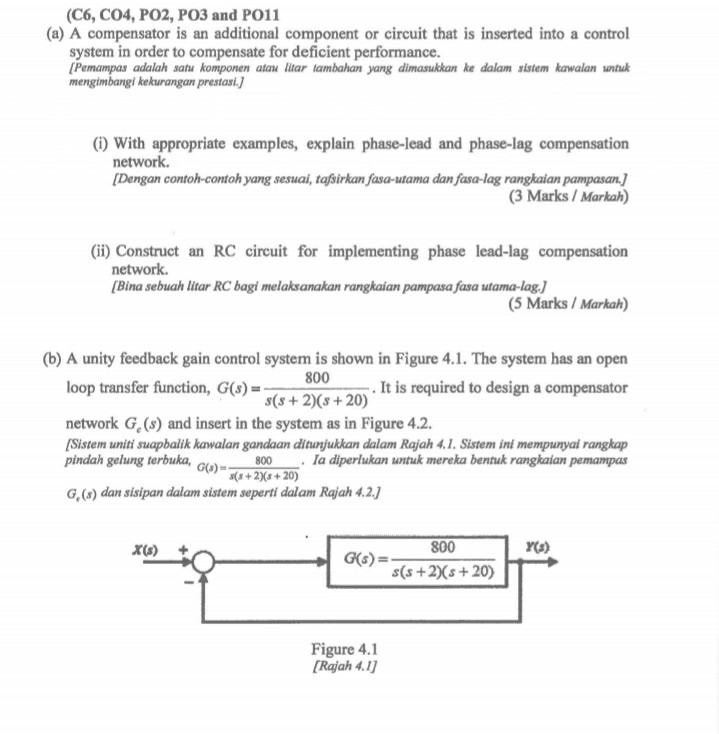modern Control system (C6, CO4, PO2, PO3 and PO11 (a) A compensator is an additional component or circuit that is inserted into a control system in order to compensate for deficient performance. Pemampas adalah satu komponen atau litar tambahan yang dimasukkan ke dalam sistem kawalan untu mengimbangi kekurangan prestasi i) With appropriate examples, explain phase-lead and phase-lag compensation network. [Dengan contoh-contoh yang sesuai, tafsirkan fasa-utama dan fasa-lag rangkaian pampasanJ (3 Marks Markah) (ii) Construct an RC circuit for implementing phase...

• ### 5. Figure 5 shows an electrical network, i) obtain the differential equations of the network and nofind the tran...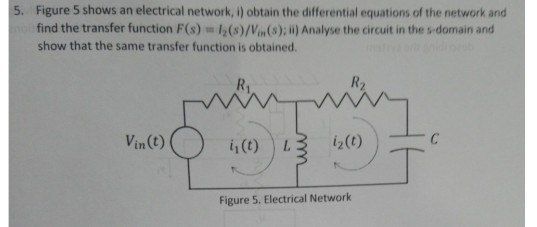5. Figure 5 shows an electrical network, i) obtain the differential equations of the network and nofind the transfer function F(s) show that the same transfer function is obtained 12(s)/Vin(s); ii) Analyse the circuit in the s-domain and R. C i2(t) Vin(t) L Figure 5. Electrical Network 4. Find the transfer function X2(s)/F(s) of the spring-mass system shown in Figure 4. The system moves over a frictionless surface. h M2 M1 Figure 4. A 2 DOF spring-mass system over a...

• ### Fall 2017 Find the transfer function, G(s) X.(s)/F(s), for the translational mechanical network shown in Figure...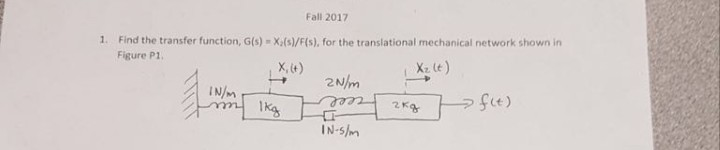Fall 2017 Find the transfer function, G(s) X.(s)/F(s), for the translational mechanical network shown in Figure P1 1. X, t) 2 N 2Ka IN-5Im

• ### CONTROL SYSTEM

A servomechanism has an open loop transfer function of G(s) = 10 / s (1+0.5s) (1+0.1s) Draw the Bode plot and determine the phase and gain margin. A networks having the transfer function (1+0.23s)/(1+0.023s) is now introduced in tandem. Determine the new gain and phase margins. Comment upon the improvement in system response caused by the network